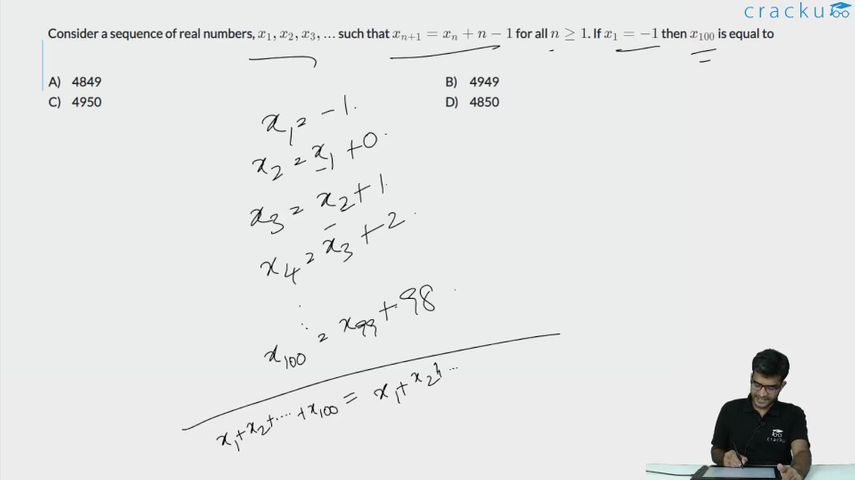Question 51

# Consider a sequence of real numbers, $$x_{1},x_{2},x_{3},...$$ such that $$x_{n+1}=x_{n}+n-1$$ for all $$n\geq1$$. If $$x_{1}=-1$$ then $$x_{100}$$ is equal to

Solution

Given $$x_{n+1}\ =\ x_n\ +\ n\ -1$$ and x1 = -1.

Considering

x1   = -1.      (1)

x2   = x1+1-1 = x1 + 0    (2)

x3   = x2 + 2 - 1  =x2 + 1     (3)

x4   = x3 + 3 - 1 = x3 + 2        (4)

x100 = x99 + 98      (100)

Adding the LHS and RHS for the hundred equations we have:

(x1+x2+......................x100) = (-1+0+.........98) + (x1+x2+...............x99)

Subtracting this we have :

(x1+...........x100) - (x1+............. x 99) = $$\frac{\left(98\cdot99\right)}{2}$$ - 1.

x100 = 4851 - 1 = 4850

Alternatively

$$x_1=-1$$

$$x_2=x_1+1-1=x_1=-1$$

$$x_3=x_2+2-1=x_2+1=-1+1=0$$

$$x_4=x_3+3-1=x_3+2=0+2=2$$

$$x_5=x_4+4-1=x_4+3=2+3=5$$

......

If we observe the series, it is a series that has a difference between the consecutive terms in an AP.

Such series are represented as $$t\left(n\right)=a+bn+cn^2$$

We need to find t(100).

t(1) = -1

a + b + c = -1

t(2) = -1

a + 2b + 4c = -1

t(3) = 0

a + 3b + 9c = 0

Solving we get,

b + 3c = 0

b + 5c = 1

c = 0.5

b = -1.5

a = 0

Now,

$$t\left(100\right)=\left(-1.5\right)100+\left(0.5\right)100^2=-150+5000=4850$$

### View Video Solution